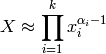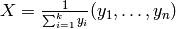# numpy.random.mtrand.RandomState.dirichlet¶

RandomState.dirichlet(alpha, size=None)

Draw samples from the Dirichlet distribution.

Draw size samples of dimension k from a Dirichlet distribution. A Dirichlet-distributed random variable can be seen as a multivariate generalization of a Beta distribution. Dirichlet pdf is the conjugate prior of a multinomial in Bayesian inference.

Parameters : alpha : array Parameter of the distribution (k dimension for sample of dimension k). size : array Number of samples to draw.

NotesUses the following property for computation: for each dimension, draw a random sample y_i from a standard gamma generator of shape alpha_i, thenis Dirichlet distributed.

References

 [R165] David McKay, “Information Theory, Inference and Learning Algorithms,” chapter 23, http://www.inference.phy.cam.ac.uk/mackay/